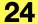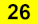## API_​AnalyticalCurveMember

Describes an Structural Analytical Model Curve Member.

```    typedef struct {
API_AnalyticalMemberType                 analyticalMemberType;
API_Guid                                 elemGuid;
API_AnalyticalCrossSection               crossSection;
API_AnalyticalCurve                      curve;
GS::Array<API_AnalyticalNode>            internalNodes;
double                                   ey;
API_Vector3D                             eyVector;
double                                   ez;
API_Vector3D                             ezVector;
API_AttributeIndex                       layerAttrIndex;
API_Analytical1DFEMType                  FEMType;
GS::Array<API_AnalyticalVaryingSegment>  varyingSegmentList;
} API_AnalyticalCurveMember;
```

#### Members

analyticalMemberTypeThe type of the represented element.
elemGuidThe unique identifier of the represented element.
crossSectionThe Structural Analytical Model Cross Section type.
curveThe curve definition.
internalNodesList of eventual Internal Nodes set on this Structural Analytical Model Curve Member.
eyThe structure eccentricity on the Y axis.
eyVectorThe Y axis of its local coordinate system.
ezThe structure eccentricity on the Z axis.
ezVectorThe Z axis of its local coordinate system.
layerAttrIndexThe containing layer’s attribute index.
FEMTypeThe FEM type of the curve member.
varyingSegmentListDescribes the profiles of the segments, if the Member represents a multisegmented Beam/Column. The sum of the span values of the segments should be equal to 1.

#### Requirements

Version: API 24 or later